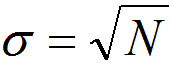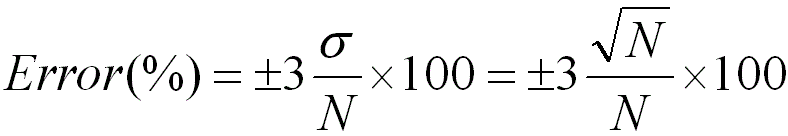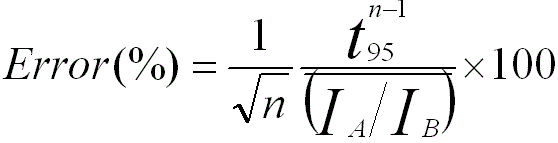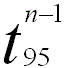Accuracy of EDS Quantification
- Practical Electron Microscopy and Database -
- An Online Book -Microanalysis | EM Book                                                                   https://www.globalsino.com/EM/

In addition to the specimen itself, the X-ray generation process is also affected by the probe size, current, and convergence angle. Fortunately, elemental concentration quantification can be done with reasonable accuracy by comparing the peak intensities with k-factors in EDS spectra. Overall, the accuracy of EDS quantification is mainly limited by the counting statistics (random errors) for the collection of X-rays not only in the determination of the kAB factors but also in the acquisition of the intensities IA,B. Therefore, the total percentage error in concentration is determined by the percentage errors of kAB and counts. In other words, the total error will be higher than the error in kAB alone or the error in counts alone.

In principle, the counting statistics of X-ray spectra limits the accuracy of quantification. If the peaks are strong comparing with a weak background, the standard deviation, σ, can be given by,----------------------------------- [2513a]
where,
N -- The number of X-ray counts in the peak after background subtraction.

For EDS and EELS techniques in EMs, if a series of measurements are repeatedly performed on a specimen, then the distribution of counts would often follow a Poisson distribution. If the number of the measurements is large, the distribution can be approximately given by a Gaussian distribution. In this case, the standard deviation of 3σ, when it has 99% confidence limit in the X-ray peak intensity, is usually used to estimate the error,----------------------------- [2513b]

Therefore, in general, the greater N is, the lower the error in the EDS analysis is.

Table 2513a lists some examples of necessary measurement counts to satisfy the needed accuracies and confidence levels.

Table 2513a. Necessary measurement counts to satisfy the needed accuracies and confidence levels.

Accuracy
Confidence level
Necessary counts
1%
99% 105
1%
68% 104

According to Equation 2513b, for a binary system with elements A and B, the error in IA/IB is given by,----------------------------- [2513c]

On the other hand, the error in IA/IB can obviously be decreased if several EDS recordings are taken. For instance, the absolute error at the 95% confidence level in n recordings of IA/IB is given by,----------------------------- [2513d]

where,-- The Student t value.-- The average value of IA/IB.

It is necessary to highlight that except for the random errors from counting statistics discussed above, some factors, however, will contribute systematic errors. Those factors are mainly:
i) The inaccuracy of the chemical composition of the standard (in this case, calibration or correction of kAB factors is needed).
ii) The spurious X-rays.
iii) The inaccuracy of evaluation of the specimen thickness.
iv) The overlapping peaks (in this case, deconvolution is needed).
v) The background (in this case, background-fitting routine is needed).

The accuracies of EDS quantitative analyses with and without standards are very different:
i) With standards:
By using standards that have compositions similar to the spcimen, the accuracy of quantitative analysis can be better than 2% for major concentrations. A more conservative value of 4-5% should be reasonable for general analysis using pure element standards. On the other hand, the accuracy also depends on the elemental concentrations. For instance, the quantitative analysis of elements with concentrations less than 5% wt. typically yields accuracies of ~10% even with standards. For specimens with rough surfaces, such as fracture spcimens or small particles, the accuracy may be as bad as 50%. Note that quantitative analysis of heterogeneous materials often provides inaccurate data since only a single standard can be used by the most common software.
ii) Without standards:
The accuracy is often much worse.

The methods of determination of k-factors are discussed on page4373 in detail.

Accurate, quantitative analysis based on EDS method can be done by the following procedure:
i) Measure the characteristic line energies corresponding to the pre-selected elements for both spcimens and standards under known operating conditions.
ii) Measure the intensity of the spectral peaks (accurate to at least 1% level in order to have ~1% of accuracy of quantification),
iii) Accurately measure the background, especially for the analysis of low concentrations (because the peak-to-background ratios are small). For instance, a 1% error is induced for a 100% error in a background measurement with a peak 100 times larger than the background, while the same error with a peak twice background introduces a ~50% error!
iv) Calculate the intensity ratios (k values).
v) Convert the values into chemical concentration.

When there are no significant differences in their X-ray absorption coefficients for the elements in the specimen, or when the specimen is thin enough, X-ray absorption corrections may be neglected to obtain an accuracy of 10-20%. However, to obtain an accuracy in the range of 5-10%, an absorption correction should be applied with an estimate of the specimen thickness.

Table 2513b lists examples of dependence of accuracy of EDS quantification on atomic number (Z) of the elements in the Periodic Table. For instance, in extremely accurate EDS quantifications, elemental detection is possible down to a few % wt. for elements with atomic numbers Z << 11.

 Table 2513b. Dependence of accuracy of EDS quantification on atomic number (Z).
Atomic number (Z)
Accuracy of EDS quantification
2≤Z << 11
A few % wt
11≤Z≤20
0.1 - 0.5 wt. %
20<<Z
0.1-0.2 wt%

Because the EDS peaks are so broad, their Gaussian tails extend over a substantial energy range, interfering with the adjacent background. Therefore, the background measurements are difficult. For a material with multiple chemical elements, the spectrum becomes more complex, and thus the quantification and interpretation is less accurate.

For the TEM configuration with a top-entry type EDS detector, the detector is placed above the objective lens in a TEM system with a high viewing angle (e.g. 70 °) to a horizontal specimen. Since X-rays entering the detector take a large take-off angle (TOA) against the specimen, a high accuracy of quantitative analysis can be obtained. However, with such configuration, the solid angle of the detector against the specimen is small, resulting in low detection efficiency.

TEM specimens can be used to simplify EDS measurements, especially quantifications, as the thin specimens avoid significant X-ray absorption and secondary x-ray fluorescence events. Those two factors typically cause significant EDS inaccuracy with bulk specimens in an electron beam microprobe or a scanning electron microscope.

Furthermore, except for the quantification inaccuracies induced by the factors mentioned above, beam damage can also introduce error. For instance, in many cases, elements are partially removed from the EM specimens (especially when TEM thin films are used) during EDS measurements (see page3123).

In summary, in order to obtain accurate elemental quantification with the experimental k-factor approach for EDS measurement, the following conditions need to be satisfied:
i) The standard and unknown samples must have the same thickness and the same bonding characteristic,
ii) Tthe spectra must be collected under identical conditions, including collection semiangle (β), probe size (d), integration window (ΔE), accelarating voltage of the incident electron beam (E0) and data aquisition time (t).

 ;